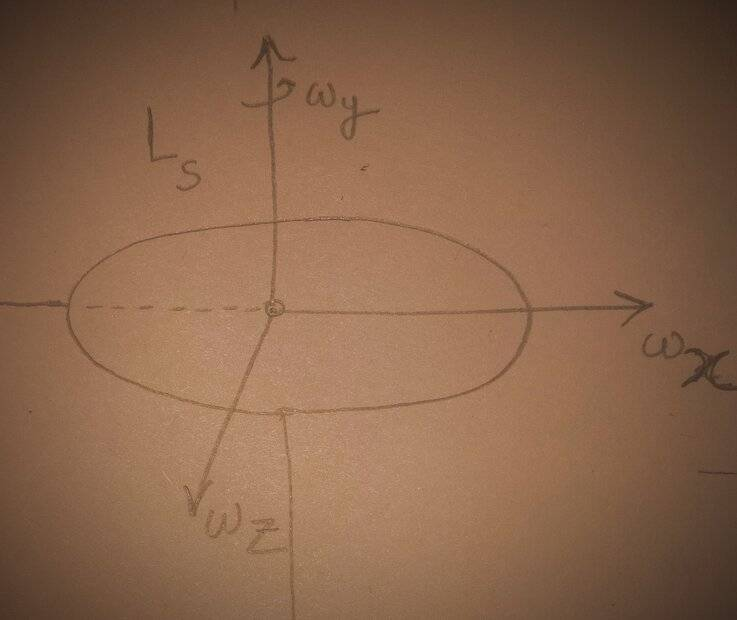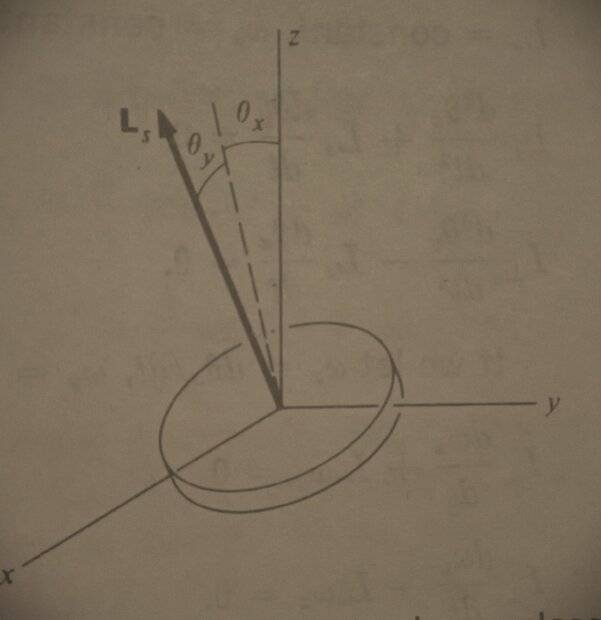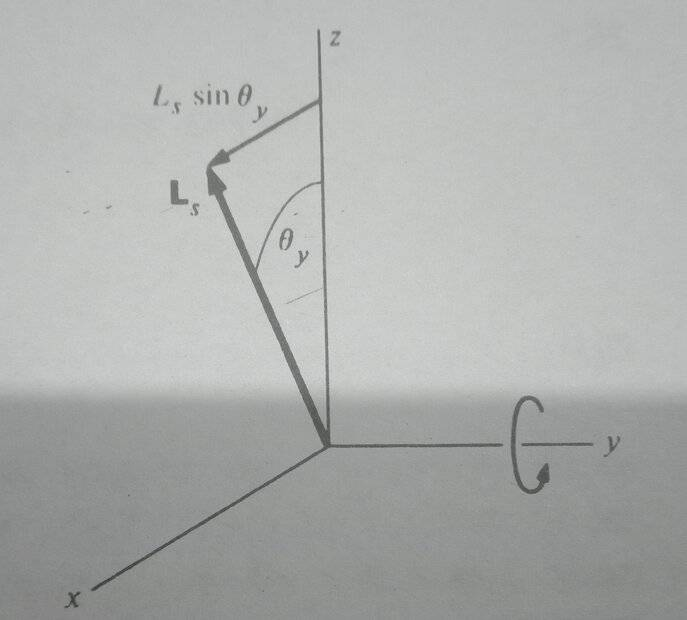# Angular momentum of a disc

• I
• Kashmir

#### Kashmir

A disc initially has angular velocities as shownIt's angular momentum along the y-axis initially is ##L_s##
I tried to find its angular momentum and ended up with this:##L=I_{x} \omega_{x}+I_{y} w_{y}+I_{z} z_{z}##

The z component of angular momentum is thus ##L_{z}=I_{z} \omega_{z}##

However I found a similar situation in a bookthat writes the components of angular momentum along x as ##L_{x}=I_{x x} \frac{d \theta_{x}}{d t}+L_{s} \sin \theta_{y}##

The book has an additional term ##L_{s} \sin \theta_{y}## for the angular momentum which I don't.

Why am I wrong ?

Last edited:
Your question is not completely clear. Perhaps the disc is initially spinning with an ## L_s ## and then given an additional rotation. Otherwise a magnetization of the disc could also make for an ## L_s ##, but in general ## L_s ## from any magnetization would be very small.

Are you asking for an expression for the angular momentum of a disk where the rotation axis is not perpendicular to the disk? Your drawing is not clear.

Are ##\omega_x, \omega_y## and ##\omega_z## the cartesian coordinates of ##\vec\omega##? How are ##I_x##, ##I_y## and ##I_z## defined? Can you describe your calculations?